# A First Demonstration of Thermodynamic Matrix Inversion

We report on the first-ever experiment towards thermodynamic artificial intelligence: solving matrix inversion problems by allowing a system of coupled electrical oscillators to thermally equilibrate with its environment.
computing
research
Author

Denis Melanson, Max Hunter Gordon, Maxwell Aifer, Kaelan Donatella, Thomas Ahle, Gavin Crooks and Patrick J. Coles

Published

November 9, 2023

Cutting-edge AI applications, like generative AI and large language models, require massive computing resources. Even today’s computing resources may not be powerful enough to unlock the full scope of applications such as probabilistic reasoning which appears to be a critical unlock towards genuine reasoning in artificial intelligence. Moreover, energy consumption is a major issue for today’s Graphical Processing Units (GPUs), with that trend only increasing with time. This motivates the search for novel computing hardware to make AI more capable, faster, and more efficient. It is worth noting that linear algebra is at the heart of machine learning and AI, and hence computing hardware for accelerating linear algebra could have a major impact on AI, so we focus on this traditional primitive for our first demonstration.

Thermodynamic computing offers a natural approach for fast, energy-efficient computations for both linear algebra and AI, as we elaborate below. The key breakthrough discussed in this blog is the first thermodynamic linear algebra experiment on a real Printed Circuit Board, namely the approximate inversion of an 8x8 matrix using a circuit of coupled electrical oscillators. This proof-of-principle demonstration is the first step towards scaling up hardware to eventually achieve thermodynamic advantage - a future goal in which thermodynamic hardware surpasses digital hardware in either speed or efficiency of computations for AI applications.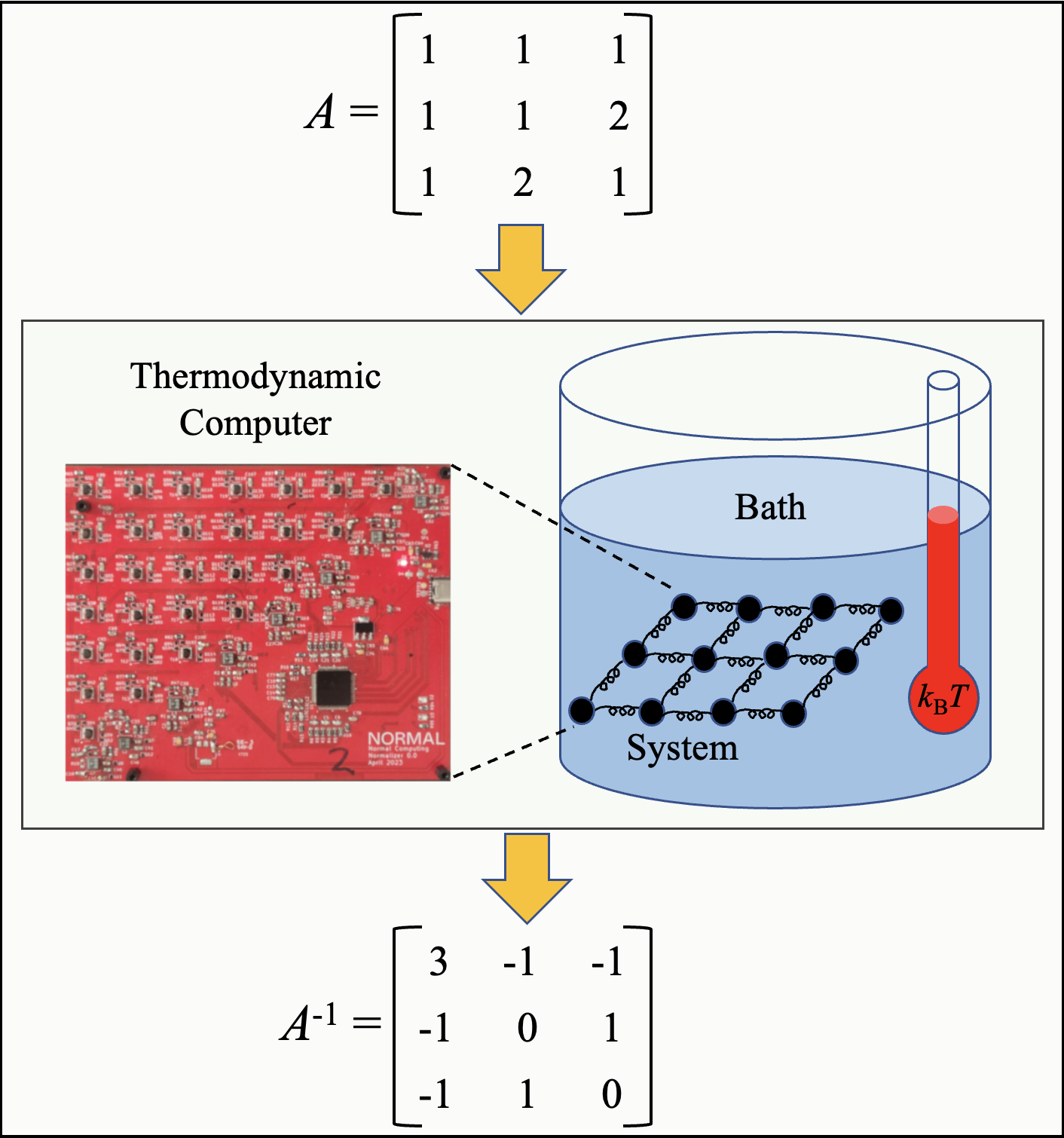Schematic diagram of thermodynamic matrix inversion. One starts by setting the couplings of a physical system to the coefficients of a matrix A. Once the system has reached thermal equilibrium, the inverse of A can be extracted from the system.

# Computing with Nature

There are many distinct approaches to computing, but one of the most energy-efficient and fast approaches is to use natural processes, i.e., physics, to perform computations. Thermodynamic processes, in particular, are well suited to computations that involve randomness, such as probabilistic AI and generative AI, as we discussed in a previous blog and paper.

Recently, we showed (somewhat surprisingly) that thermodynamic processes can also be exploited for linear algebra computations. This generated enthusiastic responses from the field, including a kind remark from Yann LeCun, as well as a question posed on Manifold Markets as to whether we will see a practical implementation of thermodynamic linear algebra in the next year. Naturally, this enthusiasm stems from the fact that linear algebra primitives, like solving linear systems of equations and computing matrix properties like the determinant and the inverse, are ubiquitous throughout engineering, science, and machine learning. Thus, having a new physics-based computing paradigm to accelerate linear algebra is exciting on multiple fronts - from reducing energy consumption, to designing new algorithms, to accelerating key applications.

# Matrix inversion algorithm

In this blog post, we report on the first-ever thermodynamic linear algebra experiment, with a specific focus on the computational primitive of matrix inversion. Matrix inversion is a subroutine in linear least squares regression, Gaussian Process Regression, Kalman filters, and some proposed extensions of transformers for language modeling. As the matrix dimension grows, it can often be the rate-limiting bottleneck of certain computations. This is largely because the time for matrix inversion scales approximately as the cube of the dimension, when performed on standard digital hardware. In contrast, a thermodynamic approach to matrix inversion (presented here) is expected to have time complexity scaling with the square of the dimension, and hence a linear speedup for thermodynamic hardware (relative to digital hardware) is predicted.

The thermodynamic algorithm for matrix inversion involves uploading the elements of the matrix to the couplings of a system of harmonic oscillators, allowing the system to come to thermal equilibrium, and then experimentally measuring the covariance matrix of the dynamical variables of the oscillators. This covariance matrix is then proportional to the inverse of the original matrix, providing the solution to the problem. The numerical simulation below (from the thermodynamic linear algebra paper) illustrates that a single trajectory maps out the inverse of a target matrix A.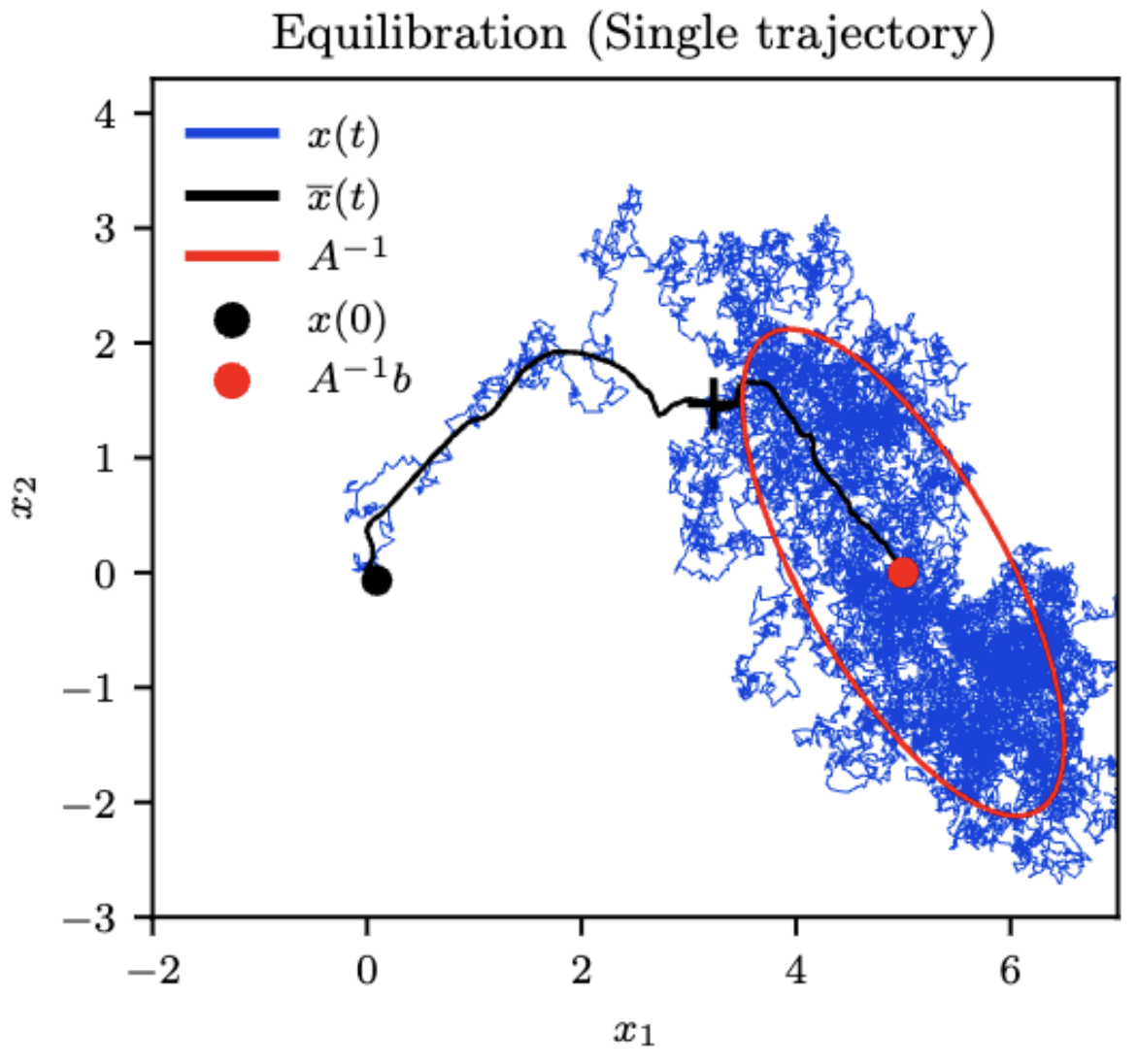Numerical simulations illustrating that a single trajectory of the system maps out the inverse of a target matrix A. Taken from the thermodynamic linear algebra paper

This is representative of the thermodynamic property of ergodicity, which means that a single trajectory of the system has the same statistical properties as a collection of many trajectories. This property allows us to collect samples from a single run instead of many separate runs, and get the same result, which can be interpreted as a form of thermodynamic parallelism. Ergodicity would not be present without the random fluctuations induced by the environment (or heat reservoir), and so it is a fundamentally thermodynamic phenomenon.

# The Thermodynamic Circuit

Our R&D team at Normal Computing designed an electrical circuit that can implement the aforementioned thermodynamic matrix inversion algorithm (among other algorithms). We refer to this circuit as a stochastic processing unit (SPU), to indicate that it uses stochasticity to facilitate the computation. This circuit is shown below, with the front (back) of the circuit board shown on the left (right). In the left panel, one can see the 8 unit cells (each cell being an LC oscillator) oriented along the diagonal, along with 28 couplings (all-to-all coupling) shown in the upper triangle. On the right panel, one can see the FPGA which is involved in uploading and downloading data from the unit cells. We have built three identical copies of the SPU, which will allow us to verify matrix inversion with three different circuits.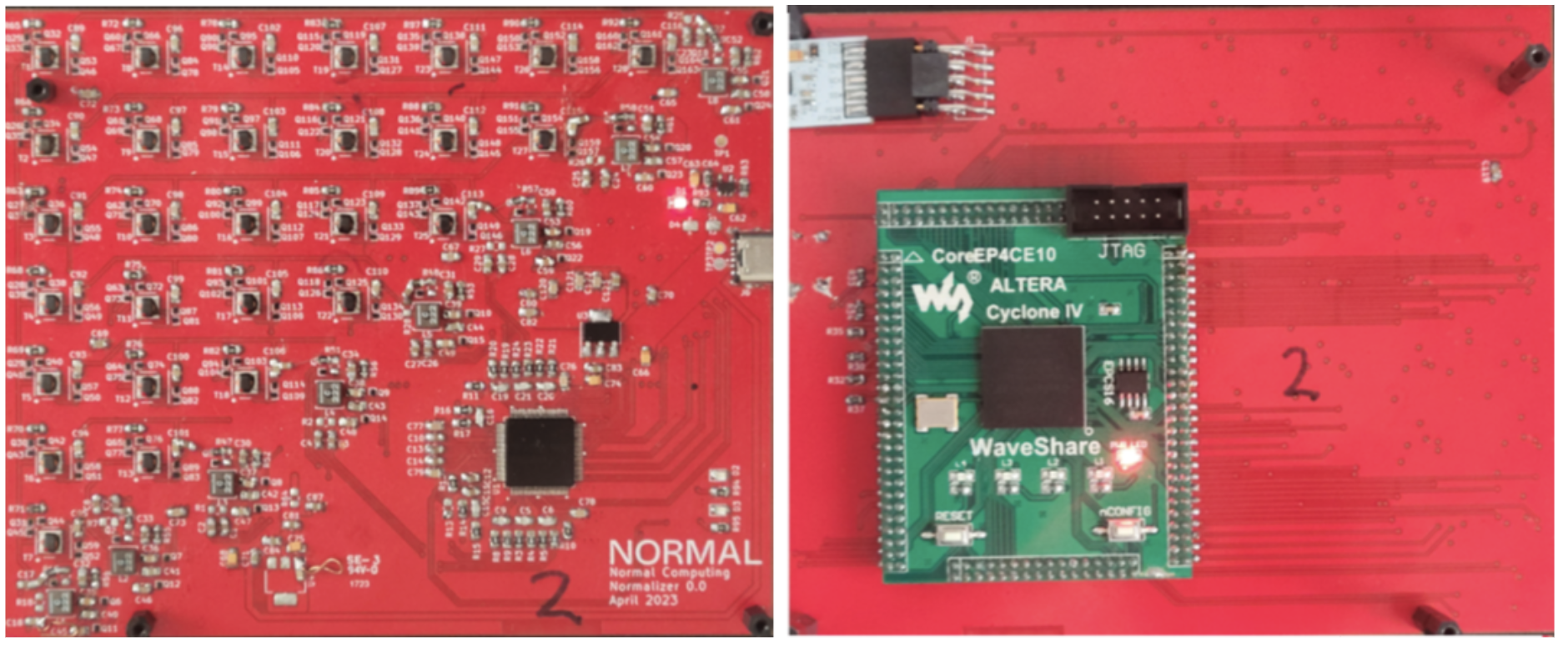Pictures of one of the SPU used in these experiments. Left: Front face of the board, featuring the unit cells and the couplings. Right: Rear face of the board, featuring the FPGA.

# The Matrix Inversion Results

For the matrix inversion experiment, we upload the matrix A of interest to the capacitance values (i.e. capacitive couplings and in-cell capacitors) in the SPU. We allow the system to come to thermal equilibrium, and then estimate the covariances of the dynamical variables to determine the matrix inverse.

## 4 x 4 Inversion

As a warmup, we first consider using only a subset of the oscillators, namely half of them, in order to invert a 4 x 4 matrix. Below we show four panels. The top left panel is the matrix inputted by the user, the top right panel is the exact inverse of the input matrix. The bottom left panel is the relative Frobenius error of the experimental results (i.e., the normalized Frobenius distance of the experimental inverse to the exact inverse) as a function of the number of samples gathered, and the bottom right panel shows the time evolution of the experimentally determined inverse as more samples are gathered. One can see that the error typically goes down with the number of samples, as expected, and the experimental inverse looks more-and-more like the true inverse as time goes on.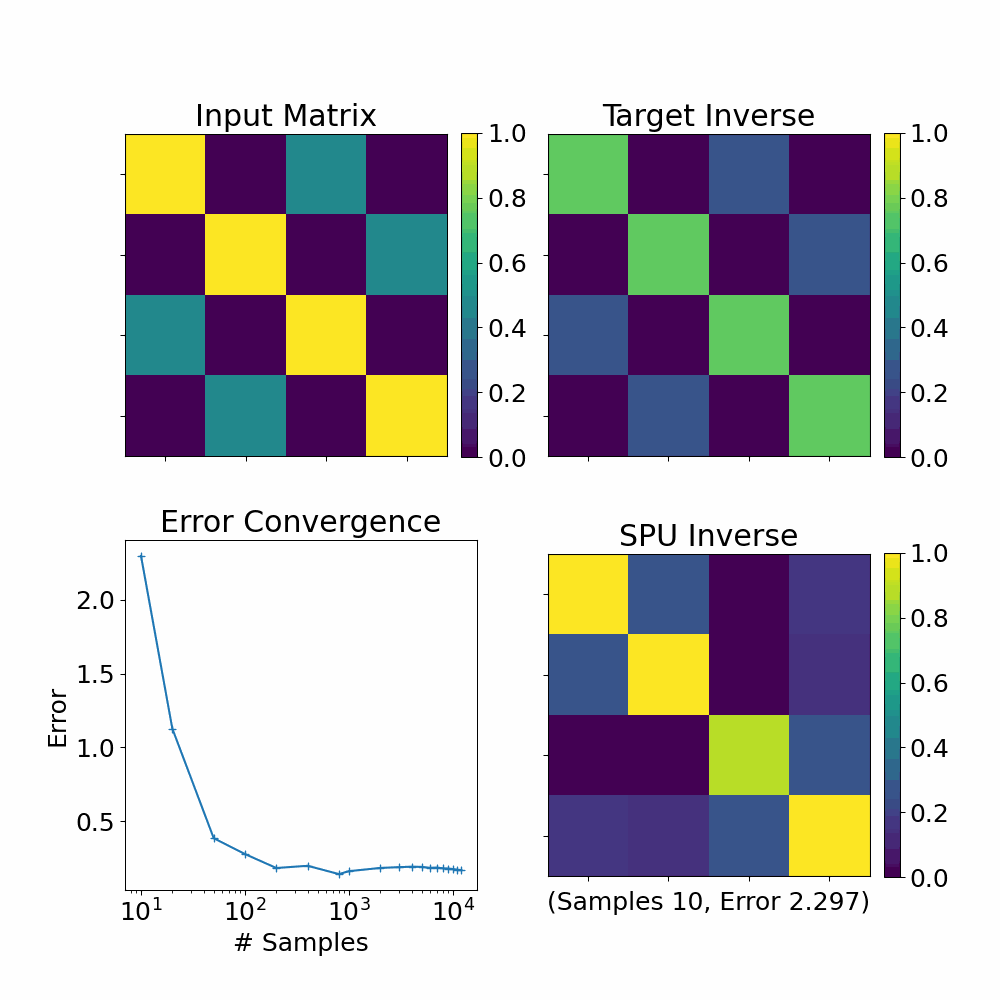Experimental results from the SPU computing the inverse of a 4 x 4 matrix. Top Left: The matrix inputted by the user. Top Right: The exact inverse of the input matrix. Bottom Left: The relative Frobenius error of the experimental results as a function of the number of samples gathered. Bottom Right: The time evolution of the experimentally determined inverse as more samples are gathered.

## 8 x 8 Inversion

Let us now consider using the entire set of oscillators, which will allow us to invert an 8 x 8 matrix. We show results on all three of our SPUs, which are identical copies of each other (up to tolerances in the component parts). Below we show six panels.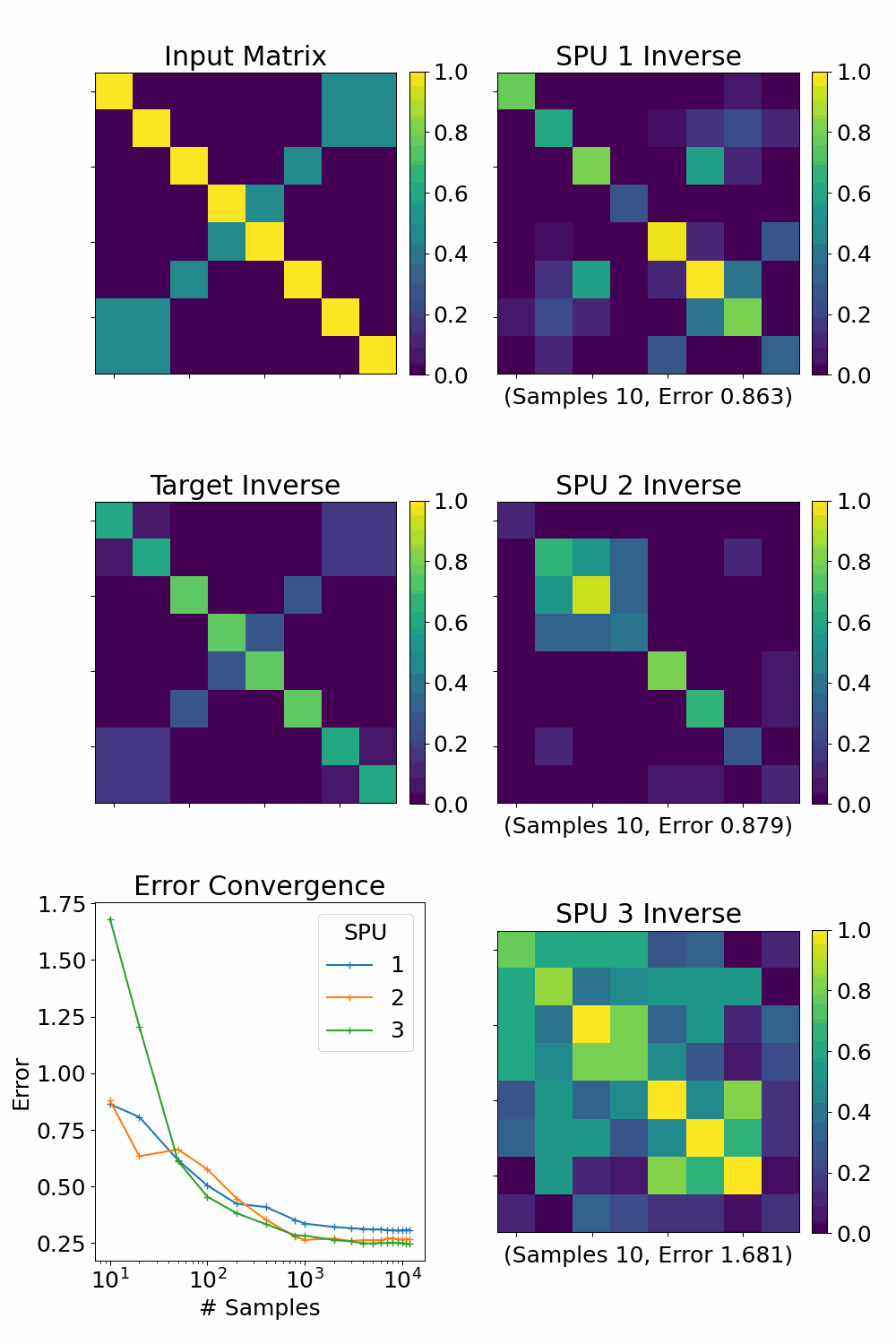Experimental results from three SPUs computing the inverse of a 8 x 8 matrix. Top Left: The input matrix. Middle Left: The true inverse. Bottom Left: The error vs number of samples for all three SPU circuits. Right Column: The time evolution of the experimental inverse for each of the three circuit boards (SPUs).

The top left shows the input matrix, the middle left shows the true inverse, and the bottom left shows the error vs number of samples for all three SPU circuits. The three panels on the right show the time evolution of the experimental inverse for each of the three circuit boards. One can see in the plot that the error tends to go down with the number of samples as expected. (The error apparently stops decreasing for large numbers of samples, which can be explained by experimental imperfections.) Moreover, one can also see from the panels on the right-hand-side that the experimentally determined inverses on all the three circuit boards tend to look visually more-and-more like the true inverse as time goes on.

# What Does the Future Hold?

The implications of these results are that thermal equilibration of simple electrical circuits can be used to perform intricate linear algebra calculations. We remark that matrices with high condition number and high dimension tend to favor performance advantage relative to state-of-the-art digital computers. This gives hints for how thermodynamic advantage will someday be achieved for matrix inversion.

We highlighted matrix inversion here, although we believe other primitives are possible, and potentially more important. While the matrix inversion algorithm is based on estimating statistical properties of a collection of samples, there are other applications of thermodynamic hardware where the output of the algorithm is the samples themselves. For example, it is often desirable to draw samples from a multivariate normal distribution, which can then be used for various tasks such as Monte Carlo simulations. Non-Gaussian distributions are increasingly used in probabilistic machine learning to model the statistical properties of real-world datasets. The preliminary success of these circuits in inverting matrices indicates that the other applications mentioned will likely also be addressable using thermodynamic hardware, which could potentially provide a computational advantage for these problems as well.

The prospect for achieving thermodynamic advantage remains an exciting future challenge. Indeed, the future is bright for exploiting thermodynamic processes for mathematical computations.

# Stay Tuned for More Details

We expect to release more details in the near future about the electronics behind these matrix inversion calculations. Be sure to sign up for updates and follow us on X.